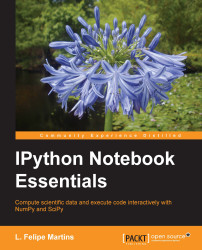•#### IPython Notebook Essentials#### Overview of this book

IPython Notebook EssentialsCreditswww.PacktPub.comPrefaceFree Chapter
A Tour of the IPython NotebookThe Notebook InterfaceGraphics with matplotlibHandling Data with pandasAdvanced Computing with SciPy, Numba, and NumbaProIPython Notebook Reference CardA Brief Review of PythonNumPy ArraysIndex## Three-dimensional plots

In this section, we present methods to display three-dimensional plots, that is, plots of mathematical objects in space. Examples include surfaces and lines that are not confined to a plate.

matplotlib has excellent support for three-dimensional plots. In this section, we will present an example of a surface plot and corresponding contour plot. The types of plot available in the three-dimensional library include wireframe plots, line plots, scatterplots, triangulated surface plots, polygon plots, and several others. The following link will help you to understand the types of plots that are not treated here: http://matplotlib.org/1.3.1/mpl_toolkits/mplot3d/tutorial.html#mplot3d-tutorial

Before we start, we need to import the three-dimensional library objects we need using the following command line:

```from mpl_toolkits.mplot3d import axes3d
```

Now, let's draw our surface plot by running the following code in a cell:

```def dist(x, y):
return sqrt(x**2 + y**2)
def fsurface...```